## Linear Methods of Applied Mathematics Evans M. Harrell II and James V. Herod*

version of 5 April 2000

(Some remarks for the instructor).

## Green functions, Fourier series, and eigenfunctions.

In this chapter we shall discuss a method for finding Green functions which makes little reference to whether a linear operator comes from an ordinary differential equation, a partial differential equation, or some other, abstract context.

In Chapter XVI we used some special one-dimensional features in order to find Green functions of ordinary differential equations. Hence we begin by reviewing which of the facts we used were connected with the one-dimensional nature of the independent variable x. We do this in tabular form.

## Facts about Green Functions and Differential Equations which are Independent of Dimensionality

1. The Green function is the integral kernel of a linear operator which inverts a differential operator L
2. The Green function solves (weakly) a differential equation of the form Lx G(x,t) =(x,t) including the boundary conditions which accompany the differential equation L y = f
3. Any two Green functions for the same differential equation (say, with different boundary conditions) have a difference which satisfies the homogeneous equation L y = 0.

On the other hand, at several points we relied on facts which, unfortunately, just won't be available for partial differential equations:

## Facts about Green Functions and ODEs which are Strictly One-Dimensional

1. The dimension of the null space of an ordinary differential operator of order n is n.
2. The solutions of a homogeneous linear ordinary differential equation satisfy a Wronskian identity.
3. The solutions to a well-posed initial-value problem for an ordinary differential equation exist and are unique. (With the method of characteristics there is an analogy for partial differential equations, but it is substantially more complicated.)
4. Thefunction can be regarded as the derivative of a step function.
5. The Green function satisfies a "jump condition" at x=t.

In this chapter our method will use infinite series to construct Green functions. It will require some special assumptions, but those can often be guaranteed whether the independent variable x is one dimensional or many dimensional. The important assumption will be that the linear operator L has a complete set of orthogonal eigenfunctions. Here completeness is meant in the sense of Chapter III. That is, we assume that there is a linear operator L such that the set of eigenvectors ek: L ek =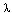k ek where <ej, ek>=0 for j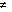k, and where any vector f in the Hilbert space can be written as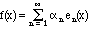.                                                             (17.1) We shall soon recall some situations where the assumptions are justified for differential operators, whether in one dimension or in many dimensions, but let us first discuss the general set-up, where we are trying to solve an equation of the form L u = f If we write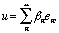(17.2) and act on both sides with the operator L, then (putting aside the question of interchanging the order of the sum and the linear operator), we find: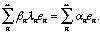Clearly, if none of the eigenvalues are 0 and there are no problems with convergence, we can solve for the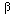n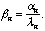Because the eigenvectors en are orthogonal, we have a formula for the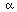n as in Chapter III: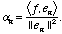If we refer again to (17.2), we get a formula for the inverse operator: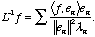(17.3) To this stage, everything is abstract. Now, let us assume that the eigenvectors are eigenfunctions, so that the inner product becomes an integral. We find: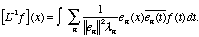The infinite sum in this expression is the kernel of an integral operator which solves L u = f - it must be the Green function!

Model Problem XVII.1. Let us use this method to solve the boundary-value problem

-u'' + u = f, u(0) = u(1) = 0.                                                             (17.4) Solution. We first ask for non-zero solutions ek(x) of the eigenvalue problem: (-D2 + I) ek(x) =k ek(x)
Boundary conditions: ek(0) = 0 = ek(1) = 0.                                     (17.5)
The solutions of the differential equation (17.5) are combinations of sin(x) and cos(x). The boundary condition ek(0) = 0 eliminates the cosines, and the other condition severely limits the possibilities for, and therefore fork: (-D2 + I) sin(k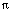x) = (k22 + 1) sin(kx). As we know from Chapter VI, these sine functions are a complete orthogonal set. That is, any square-integrable function F(x) can be written as a Fourier sine series in terms of these functions.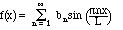According to the ideas of this chapter, the solution of (17.4) is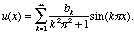(17.6)

Because of the formula for the coefficients bk, we see that the Green function is: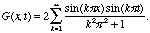(17.7)
As mentioned, this method makes little reference to the fact that the L of Model Problem XVII.1 is an ordinary differential operator.

Let us consider the same problem from a different point of view, by expanding the delta function in a Fourier sine series. Bravely applying the usual formulae for the Fourier sine series to the delta function, we find that for 0 < x < 1,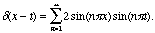In other words, if the function f(x) above happens to be the delta function, then bn = 2 sin(nt). If we now use (17.6), we get the same formula (17.7) for the Green function.

Let us now turn our attention to some partial differential equations which use the Laplace operator, introduced in Chapter IX. We begin with Laplace's equation (9.1-2):

Model Problem XVII.2. Let us use the same method to solve the two-dimensional boundary-value problem for 0 < x1, x2 < 1,

-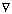2 u = f(x),                                                             (17.8)
Boundary conditions: u = 0 when either x1 = 0 or 1 or x2 = 0 or 1.
Solution. We can solve this problem with a double Fourier sine series. The reason for this is that the sine functions fjk(x) := sin(jx1) sin(kx2) are not only a complete set, but also have a special relationship to the Laplace operator, as eigenfunctions (including the correct boundary conditions): -2 fjk(x) =jk fjk(x), wherejk := (j2 + k2). As remarked above, we may derive the series for the Green function by treating it as a classical function and finding its Fourier series by exploiting the equation (17.8) with f(x) =(x-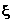) for any given vector.

Since(n)(x -) =(x1-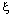1)(x2-2) (see the Exercises), The Fourier series for the two-dimensional delta function is likewise a product: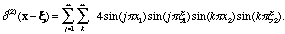At the same time, if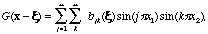then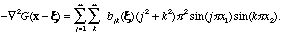By comparing these equations, we determine the coefficients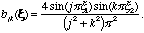Exercises XVII
1. In Exercises 5, 13, 14, and 19 of Chapter 16, you constructed Green functions for different boundary conditions but the same differential equation of the form LxG = f. Verify in each such case that if you define y := G(a)(x,t) - G(b)(x,t), then y solves the homogeneous differential equation Lxy = 0.
2. The Green function of Model Problem XVII.1 must be the same as the one you found in Exercise 10 (d) of Chapter XVI, despite looking entirely different. Show that the two Green functions are equal.
3. Using the definition of the delta function, show that if(n)(x -) denotes the delta function in n dimensions, then(n)(x -) =(x1-1)(x2-2)...(xn-n).
4. Use the Fourier cosine series to find the Green function for the boundary-value problem -2 u + u = f(x),                                                             (17.9)
Boundary conditions of Neumann type: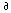u/x1 = 0 when x1 = 0 or 1, andu/x2 = 0 when x2 = 0 or 1.
Note: The addition of u in (17.9) prevents the problem from being in the second Fredholm alternative. SHow that if combine the Neumann boundary conditions of this problem with the equation in (17.8), then the second alternative applies.
5. Solve the Poisson equation (17.8) on the rectangle 0 < x1 < 2, 0 < x2 < 1, with Dirichlet (u=0) boundary conditions on the boundary.
6. Solve the Poisson equation (17.8) on the rectangle 0 < x1 < 2, 0 < x2 < 1, with Dirichlet (u=0) boundary conditions at x1 = 0 and 2, but Neumann boundary conditions at x2 = 0 and 1.
7. Solve the Poisson equation (17.8) on the rectangle 0 < x1 < 2, 0 < x2 < 1, with Dirichlet (u=0) boundary conditions at x1 = 0 and x2 = 0, but Neumann boundary conditions at x1 = 2 and x2 = 1. Note: First figure out what the eigenfunctions are which satisfy the boundary conditions. The spectral theorem guarantees that they are a complete orthogonal set.